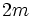# Involutions are either conjugate or have an involution centralizing both of them

This article gives the statement, and possibly proof, of a statement where the conclusion of the statement involves a disjunction (OR) of two possibilities. The prototypical form is: "every A is a B or a C."

## Statement

Suppose$G$ is a finite group and$x,y$ are two involutions of$G$. Then, at least one of these two is true:

1.$x$ and$y$ are in the same conjugacy class.
2. There exists an involution$z$ centralizing both$x$ and$y$.

## Facts used

1. Dihedral trick

## Proof

Given: A finite group$G$, two involutions$x,y$ of$G$.

To prove: Either$x$ or$y$ are conjugate or there is an involution centralizing both of them.

Proof: By fact (1),$\langle x,y \rangle$ is a dihedral group of order$2m$, where$m$ is the order of$xy$. This dihedral group has$xy$ generating a cyclic subgroup of order$m$ and$x$ acts on this by inverting$xy$.

If$m$ is odd, then$x,y$ are conjugate in$\langle x,y \rangle$, hence in$G$. Otherwise,$m$ is even, in which case the element$z = (xy)^{m/2}$ is an involution centralizing both$x$ and$y$.